library(hbm4ecology)
library(rjags)
library(tidyverse)
library(posterior)

The main idea is to link the capture-mark-recapture (CMR) models for yearly observations together by a hierarchical structure. In this case study inspired by the article of Rivot and Prévost (2002), the years are the statistical units that look alike and that hypothesized resemblance allows for transferring information from a given year to the other years.

## Data

Relatively long but sparse (small sample size) series of data are quite common when dealing with CMR surveys aimed at estimating the abundance of wild populations over a series of years. For instance on the Oir River, the rangers from the French National Research Institute for Agronomy (INRA) and from the National Office of Water Management (ONEMA) have collected CMR data about adult salmon that swam back to spawn in the Oir River for the years 1984–2000.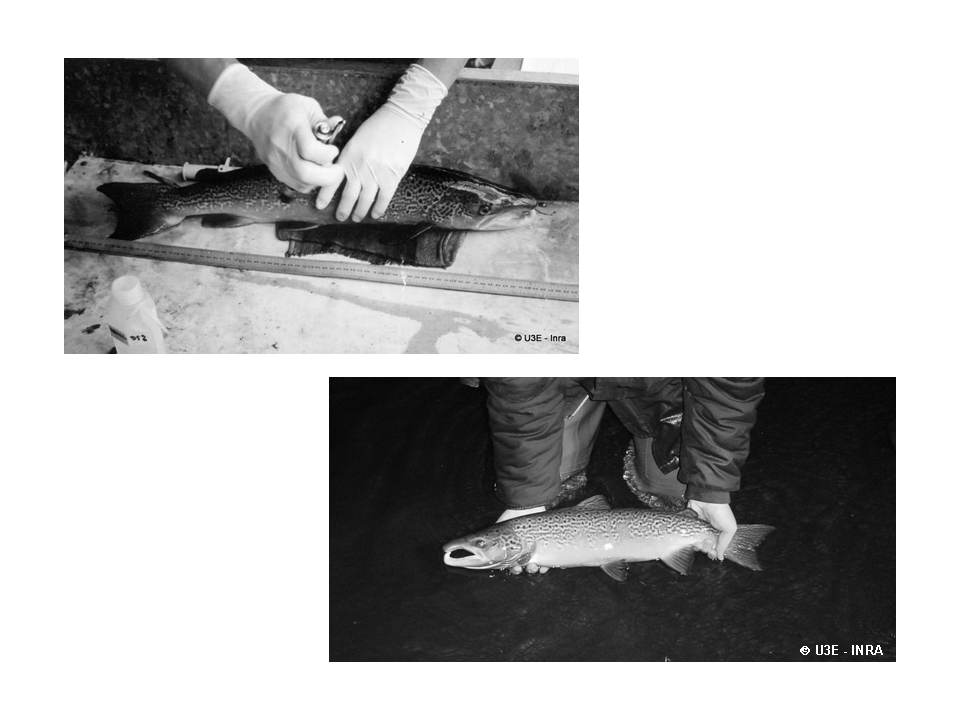Figure 1 – Marking a spawner entering the Scorff River

data("CmrOir")
CmrOir[2:7] %>% as_tibble() %>%
mutate(Year = 1984:2000, .before=1)%>% knitr::kable()
Year y1 y2 y3 y4 y5 y6
1984 167 10 3 154 12 10
1985 264 37 11 216 21 4
1986 130 28 9 93 5 4
1987 16 3 1 12 2 22
1988 226 35 8 183 12 0
1989 235 31 5 199 56 0
1990 15 4 4 7 2 15
1991 44 0 0 44 23 1
1992 31 10 1 20 4 5
1993 100 17 2 81 4 3
1994 32 12 2 18 1 4
1995 109 6 1 102 39 7
1996 70 13 2 55 25 57
1997 56 19 3 34 12 3
1998 34 3 1 30 6 30
1999 154 5 1 148 23 12
2000 53 0 0 53 4 33

For each year $$t$$ from $$1984$$ to $$2000$$, $$y_{1,t}$$ denotes the number of fish trapped at the Cerisel station (close to the mouth of the river). $$y_{2,t}+y_{3,t}$$ individuals from the captured ones are not replaced upstream, either because they died during manipulation or because they are removed for experimental use or for hatchery production. Let $$y_{4,t}=y_{1,t}-(y_{2,t}+y_{3,t})$$ the number of (tagged) fish released. These spawners are individually marked before they keep on swimming upstream . The recapture sample is gathered during and after spawning (see more details on recapture conditions hereafter). Let us denote as $$y_{5,t}$$ and $$y_{6,t}$$ the number of marked and unmarked fish among recaptured fish, respectively.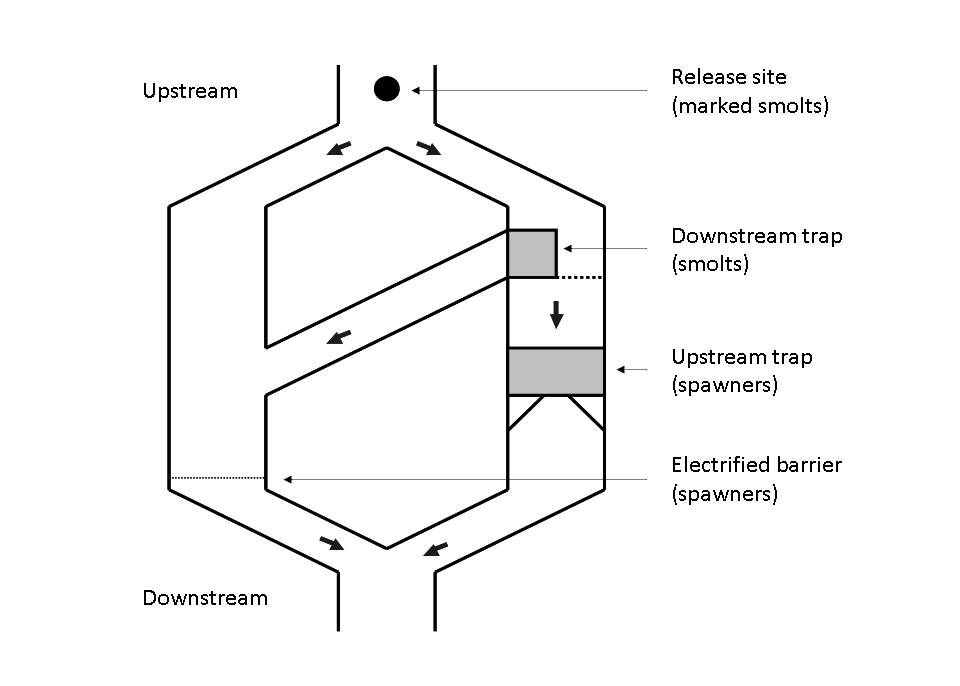Figure 2 – Scheme of the Cerisel trapping facility (Oir river, Normandy, France) working as a double downstream (for smolt runs) and upstream (for spawner runs) partial counting fence. The black circles at the top of the figure indicates the release site of tagged smolts and spawners. The release site and the downstream trap are 1 km away from each other.

## Modeling

### Observation submodels for the first phace (Cerisel trapping place)

Let,

• $$\nu_t$$ be the population size of spawners at year $$t$$
• $$\pi_t^1$$ be the unknown trapping efficiency,

and assume all of the $$\nu_t$$ spawners are independently and equally catchable in the trop with a probability $$\pi^1_t$$ constant over the migration season. Then, the number of fish trrapped at the counting fence during migration time is the result of a Binomial experiment:

• $$y_{1,t} \sim Binomial(\nu_t, \pi^1_t)$$.

### Observation submodels for the second phase (re-collection during and after spawning)

The recapture sample is obtrainged by three methods: electrofishing on the spawning grounds, collection of dead fish after spawning, and trapping of spent fish at the downstram trap of the Cerisel facility. Modeling recapture with Binomial experiments with effeciency $$\pi^2_t$$ is reasonable assuming the three following hypotheses: No spawner runs downstream after getting over the trap ($$H1$$); there is no tag shedding ($$H2$$); the recapture probability $$\pi_{t}^{2}$$ is the same for all the fish whether or not marked ($$H3$$):

• $$y_{5,t} \sim Binomial(y_{4,t}, \pi^2_t)$$
• $$y_{6,t} \sim Binomial(\nu_t - y_{1,t}, \pi^2_t)$$

### Latent layers

Let $$Z_t = (\nu_t, \pi^1_t, \pi^2_t)$$ be the latent vector and $$y_t = (y_{1,t}, y_{5,t}, y_{6,t})$$ the observations. The natural choice for the latent distributions are the Beta distribtion for $$\pi^1_t$$ and $$\pi^2_t$$ and the Negative Binomial ditribution for $$\nu_t$$:

• $$\pi^1_t \sim Beta(a_1, b_1)$$
• $$\pi^2_t \sim Beta(a_2, b_2)$$
• $$\nu_t \sim NegBinomial(c,d)$$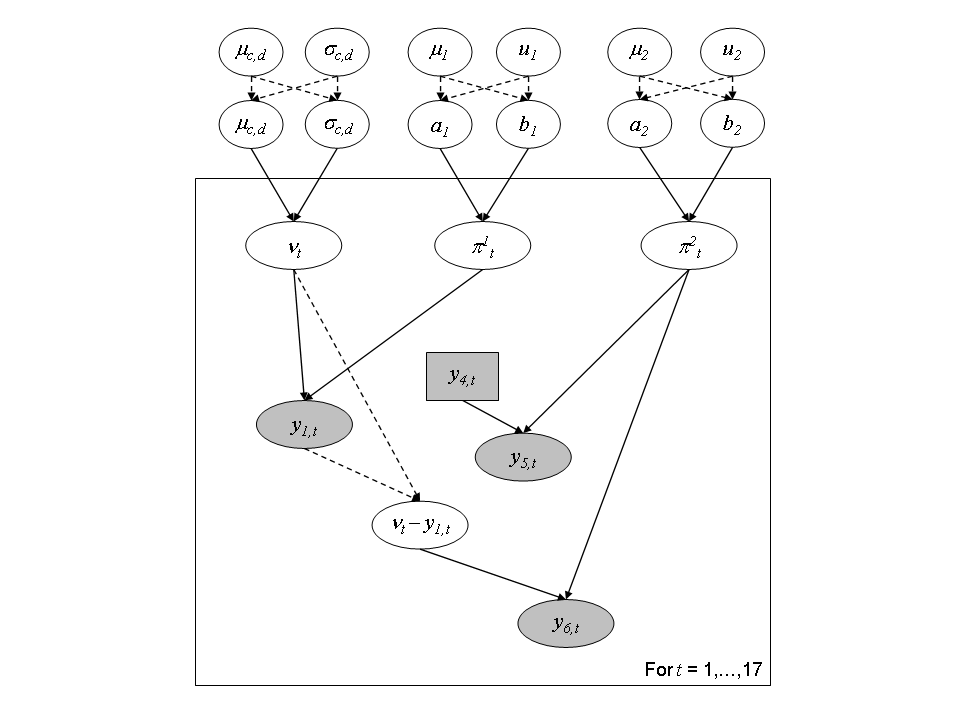Figure 3 – Directed Acyclic Graph representation of the hierarchical structure for the joint modeling of capture-mark-recapture experiments for the 17 years.

We assign a diffuse prior distribution to the higher level parameters $$\theta = (a_1, a_2, b_1, b_2, c, d)$$ to reflect some ignorance about them. We use the following transform which recovers well understood meaning. For the trapping efficiency:

• $$\mu_{a,b} = \frac{a}{a+b}$$ is the mean of the Beta distribution, with $$\mu_{a,b} \sim Beta(1.5, 1.5)$$
• $$u_{a,b} = a+b$$ can be interpreted as a prior sample size that scale the variance of the Beta distribution, with $$\log(u_{a,b} \sim Uniform(0,10)$$).

For the number of spawners:

• $$\mu_{c,d} = \frac{c}{d}$$ is the mean of the Negative Binomial distribution, with $$\mu_{c,d} \sim Uniform(0, \mu_{max})$$, where $$\mu_{max} = 3000$$ fish.
• $$\sigma^2_{c,d} = \frac{c(d+1)}{d^2}$$ is the variance of the Negative Binomial distrution, with $$\log(\sigma_{c,d}) \sim Uniform(\log(\mu_{c,d}), \log(\sigma^2_{max}))$$, where $$\sigma^2_{max} = 12$$ since we do not believe that the standard deviation might exceed $$400$$ fish.

### Independent modeling

To show how a transfer of information between years is organized by the hierarchical model, we compare its results with the model assuming independence between years. For the models with independence, independent prior distributions with known parameters were set on $$(\nu_{t},\pi_{t}% ^{1},\pi_{t}^{2})$$:

• $$\pi_{t}^{1} \; \sim \; Beta(1.5,1.5)$$
• $$\pi_{t}^{2} \; \sim \; Beta(1.5,1.5)$$
• $$\nu_{t} \; \sim \; Uniform(1,3000)$$

## Implementation, results and analysis

### Independent model

The model can be written in rjags as the following string:

ind_model_str <- "
model {

# N = 17 years (1984 to 2000)
pnu <- rep(1, 3000)
for (i in 1:N)
{

# Prior for trapping efficiency pi1

pi1[i] ~ dbeta(1.5,1.5)

# Prior for recapture efficiency pi2

pi2[i] ~ dbeta(1.5,1.5)
nu[i] ~ dcat(pnu[])

# Escapement (number of fish that mate)

Nsp[i] <- max(nu[i]-y2[i]-y3[i],0)

# Number of unmarked fish

nm[i] <- max(nu[i]-y1[i],0)

#  Likelihood (binomial for capture and recapture)

y1[i] ~ dbin(pi1[i],nu[i])
y6[i] ~ dbin(pi2[i],nm[i])
y5[i] ~ dbin(pi2[i],y4[i])

} # end of loop on year i

} # end of model"

And we fix the following initialization points for each of the three chains.

ind_init <-
list(
list(pi1 = rep(.5,CmrOir$N), pi2 = rep(.5, CmrOir$N), nu = rep(300, CmrOir$N)), list(pi1 = rep(.2,CmrOir$N), pi2 = rep(.5, CmrOir$N), nu = rep(500, CmrOir$N)),
list(pi1 = rep(.8,CmrOir$N), pi2 = rep(.5, CmrOir$N), nu = rep(1000, CmrOir$N)) ) ind_model <- jags.model(file = textConnection(ind_model_str), data = CmrOir, inits = ind_init, n.chains = 3) #> Compiling model graph #> Resolving undeclared variables #> Allocating nodes #> Graph information: #> Observed stochastic nodes: 51 #> Unobserved stochastic nodes: 51 #> Total graph size: 244 #> #> Initializing model # Inferences update(ind_model, n.iter = 10000) posterior_sample_ind <- coda.samples(ind_model, variable.names = c("pi1", "pi2", "nu"), n.iter = 10000, thin = 10) #### Diagnostic summarise_draws(as_draws_df(posterior_sample_ind), default_convergence_measures()) %>% knitr::kable() variable rhat ess_bulk ess_tail nu 1.0013690 993.8650 1402.8073 nu 1.0019188 1301.0693 1817.1009 nu 1.0047103 696.9189 939.2453 nu 1.0043067 1093.5905 1601.1423 nu 1.0019027 1022.9990 1278.6559 nu 1.0004007 2659.7480 2936.2192 nu 1.0004199 1561.2548 1785.0422 nu 0.9998430 2682.8475 2880.7041 nu 1.0002507 2026.1694 2364.0482 nu 1.0007880 835.2781 1192.8109 nu 1.0008658 1139.6212 1296.5161 nu 1.0007640 2835.5706 2970.8976 nu 0.9997233 2644.7484 2416.3082 nu 1.0015857 2567.8427 2769.8569 nu 1.0000188 1724.8200 2041.7691 nu 0.9998513 2208.8187 2771.5664 nu 1.0047596 852.9044 1214.2785 pi1 1.0013372 974.9461 1425.9177 pi1 1.0017211 1288.5764 1998.3713 pi1 1.0041901 716.0069 969.2572 pi1 1.0036057 1248.7285 1520.3286 pi1 1.0020058 1027.5631 1278.2841 pi1 1.0000219 2686.6746 2945.4777 pi1 1.0011686 1667.8347 1673.9751 pi1 1.0017163 2986.1406 2824.9457 pi1 1.0006148 2074.6710 2481.0897 pi1 1.0011731 847.9701 1228.4709 pi1 1.0006020 1091.6438 1381.1840 pi1 1.0000989 2970.5276 2988.4496 pi1 1.0002324 2717.8513 2878.4232 pi1 0.9996568 2805.3258 2829.3109 pi1 1.0004164 1871.1486 2035.4621 pi1 0.9996745 2209.9208 2755.1743 pi1 1.0043925 867.3266 1249.9307 pi2 1.0016015 1406.6701 2134.6575 pi2 1.0004181 2505.9535 2771.2567 pi2 1.0003594 1250.1963 1850.7094 pi2 1.0053972 1091.9978 1696.1680 pi2 1.0003103 2565.7296 2791.5070 pi2 0.9999579 2652.4572 2733.7803 pi2 1.0006617 1645.5332 2024.3918 pi2 1.0005218 2879.1677 2839.1901 pi2 1.0008550 2117.5513 2412.4405 pi2 1.0008445 1471.3449 2052.5595 pi2 1.0001669 1418.7802 2134.3629 pi2 1.0015196 2949.2289 2616.5255 pi2 1.0001725 2711.4697 2908.0769 pi2 0.9996217 2993.4374 2988.8836 pi2 1.0007358 1865.2145 2110.1324 pi2 1.0006582 2660.0672 2577.1729 pi2 1.0033700 920.0852 1325.1539 ### Hierarchical model hier_model_str <- " model { # N = 17 years (1984 to 2000) # Hyperprior for thetrapping efficiency mu1_ab ~ dbeta(1.5, 1.5) log_u1_ab ~ dunif(0,10) u1_ab <- exp(log_u1_ab) alpha1 <- mu1_ab * u1_ab beta1 <- (1-mu1_ab)*u1_ab # Hyperprior for the recapture efficiency mu2_ab ~ dbeta(1.5, 1.5) log_u2_ab ~ dunif(0,10) u2_ab <- exp(log_u2_ab) alpha2 <- mu2_ab * u2_ab beta2 <- (1-mu2_ab)*u2_ab # Hyperperior for nu_max pmunu <- rep(1, 3000) mu_nu ~ dcat(pmunu) inf_log_sig2_nu <- log(mu_nu) log_sig2_nu ~ dunif(inf_log_sig2_nu, 12) sig2_nu <- exp(log_sig2_nu) p <- mu_nu/sig2_nu # d = p/(1-p) c <- mu_nu * p*(1-p) # Predictive distributions pi1_pred ~ dbeta(alpha1, beta1) pi2_pred ~ dbeta(alpha2, beta2) nu_pred ~ dnegbin(p,c) for (i in 1:N) { # Prior for trapping efficiency pi1 pi1[i] ~ dbeta(alpha1, beta1) # Prior for recapture efficiency pi2 pi2[i] ~ dbeta(alpha2, beta2) nu[i] ~ dnegbin(p,c) # Escapement (number of fish that mate) Nsp[i] <- max(nu[i]-y2[i]-y3[i],0) # Number of unmarked fish nm[i] <- max(nu[i]-y1[i],0) # Likelihood (binomial for capture and recapture) y1[i] ~ dbin(pi1[i],nu[i]) y6[i] ~ dbin(pi2[i],nm[i]) y5[i] ~ dbin(pi2[i],y4[i]) } # end of loop on year i } # end of model" And we fix the following initialization points for each of the three chains. hier_init <- list( list(pi1 = rep(.5, CmrOir$N), pi1_pred = .5, mu1_ab = .6,  log_u1_ab = .6,
pi2 = rep(.5, CmrOir$N), pi2_pred = .2, mu2_ab = .6, log_u2_ab = .6, nu = rep(300, CmrOir$N), nu_pred = 300, mu_nu = 10, log_sig2_nu = 3),
list(pi1 = rep(.2, CmrOir$N), pi1_pred = .2, mu1_ab = .5, log_u1_ab = .25, pi2 = rep(.5, CmrOir$N), pi2_pred = .2, mu2_ab = .5,  log_u2_ab = .25,
nu = rep(500, CmrOir$N), nu_pred = 500, mu_nu = 50, log_sig2_nu = 4), list(pi1 = rep(.8, CmrOir$N), pi1_pred = .5, mu1_ab = .5,  log_u1_ab = .25,
pi2 = rep(.5, CmrOir$N), pi2_pred = .5, mu2_ab = .5, log_u2_ab = .25, nu = rep(1000, CmrOir$N), nu_pred = 1000, mu_nu = 100, log_sig2_nu = 5)
)
hier_model <- jags.model(file = textConnection(hier_model_str),
data = CmrOir, inits = hier_init,
n.chains = 3)
#> Compiling model graph
#>    Resolving undeclared variables
#>    Allocating nodes
#> Graph information:
#>    Observed stochastic nodes: 51
#>    Unobserved stochastic nodes: 60
#>    Total graph size: 268
#>
#> Initializing model

# Inferences
pars <-  c("pi1", "pi1_pred", "mu1_ab", "u1_ab",
"pi2", "pi2_pred", "mu2_ab", "u2_ab",
"nu", "nu_pred", "mu_nu", "log_sig2_nu")

update(hier_model, n.iter = 10000)
posterior_sample_hier <- coda.samples(hier_model,
variable.names = pars,
n.iter = 10000,
thin = 10)

#### Diagnostic

summarise_draws(as_draws_df(posterior_sample_hier),
default_convergence_measures()) %>%
knitr::kable()
variable rhat ess_bulk ess_tail
log_sig2_nu 0.9997319 2395.384 2669.261
mu1_ab 1.0019660 2661.879 2704.381
mu2_ab 0.9996043 2624.235 2893.343
mu_nu 0.9994781 2275.223 2604.974
nu 1.0011896 1554.654 1908.692
nu 1.0021367 1624.971 2254.885
nu 1.0030374 1050.078 1679.898
nu 1.0009683 1299.503 2230.798
nu 1.0000910 1246.117 1293.924
nu 0.9996345 2522.676 2617.274
nu 1.0005535 1588.662 2498.688
nu 1.0003906 2945.202 2953.627
nu 1.0012364 1353.796 2203.618
nu 1.0018569 1230.897 1571.830
nu 1.0015666 1267.747 2095.106
nu 1.0001599 3007.668 2586.552
nu 0.9994760 2569.897 2742.954
nu 1.0003968 2675.193 2313.388
nu 1.0023591 2018.946 2427.733
nu 0.9996841 2130.689 2468.704
nu 1.0027445 1627.417 2203.366
nu_pred 1.0015837 2746.470 2720.931
pi1 1.0016010 1528.499 2088.597
pi1 1.0033526 1587.426 2098.956
pi1 1.0029123 1115.654 1764.632
pi1 1.0002038 1625.597 2489.303
pi1 0.9995230 1302.381 1359.398
pi1 0.9997740 2623.877 2829.082
pi1 1.0016581 1575.385 2229.445
pi1 0.9999254 2925.307 2878.503
pi1 1.0008627 1376.934 2317.700
pi1 1.0011772 1229.514 1726.110
pi1 1.0023860 1263.814 1940.431
pi1 1.0010282 3063.732 2677.979
pi1 0.9999112 2713.748 2455.949
pi1 1.0011365 2372.484 2448.762
pi1 1.0000181 1931.741 2721.123
pi1 1.0002541 2235.443 2666.400
pi1 1.0001212 1817.507 2298.095
pi1_pred 1.0002161 2665.024 3040.714
pi2 1.0005623 2214.258 2484.804
pi2 1.0013452 2659.608 2688.503
pi2 1.0027861 1455.231 2294.388
pi2 1.0000595 1535.814 2356.742
pi2 1.0002026 2820.090 2905.713
pi2 1.0000021 2674.456 2430.697
pi2 1.0006700 1801.042 2519.803
pi2 1.0012373 2720.970 2740.133
pi2 1.0006231 1773.399 2573.889
pi2 1.0006133 2130.209 2412.496
pi2 1.0017309 1702.560 2417.880
pi2 0.9999596 2952.623 2928.377
pi2 0.9995414 2474.594 2684.712
pi2 1.0006674 2592.162 2387.218
pi2 1.0004399 2032.824 2570.932
pi2 1.0003019 2608.952 2624.330
pi2 1.0010658 1840.344 2480.820
pi2_pred 0.9993152 3125.068 3077.635
u1_ab 0.9993884 2650.360 2852.368
u2_ab 1.0000074 2462.765 2983.584

### Results and analysis

We extract the results of both model into long form and wide form data frames.

dfl_ind <- extract_longer(posterior_sample_ind) %>%
mutate(Model = "Independent")
dfw_ind <- extract_wider(posterior_sample_ind) %>%
mutate(Model = "Independent")
dfl_hier <- extract_longer(posterior_sample_hier) %>%
mutate(Model = "Hierarchical")
dfw_hier <- extract_wider(posterior_sample_hier) %>%
mutate(Model = "Hierarchical")

#### Comparing the efficiency and number of spawners distribution for both models

dfl_ind %>% bind_rows(dfl_hier) %>%
filter(str_detect(parameter, "^pi1\\.")) %>%
mutate(Years = as.factor(seq(1984,2000)[as.integer(str_sub(parameter, 5))])) %>%  # 5 = length("pi1.") + 1
ggplot() +
aes(x = Years, y = value, fill = Model) +
geom_boxplot(outlier.shape = NA, outlier.size = .25) +
scale_x_discrete(guide = guide_axis(angle = 90)) +
ylim(c(0,1)) +
scale_fill_manual(values = c("gray50", "gray90")) +
ylab("Trapping efficiency") + xlab("Years") +
theme_classic(base_size = 15L)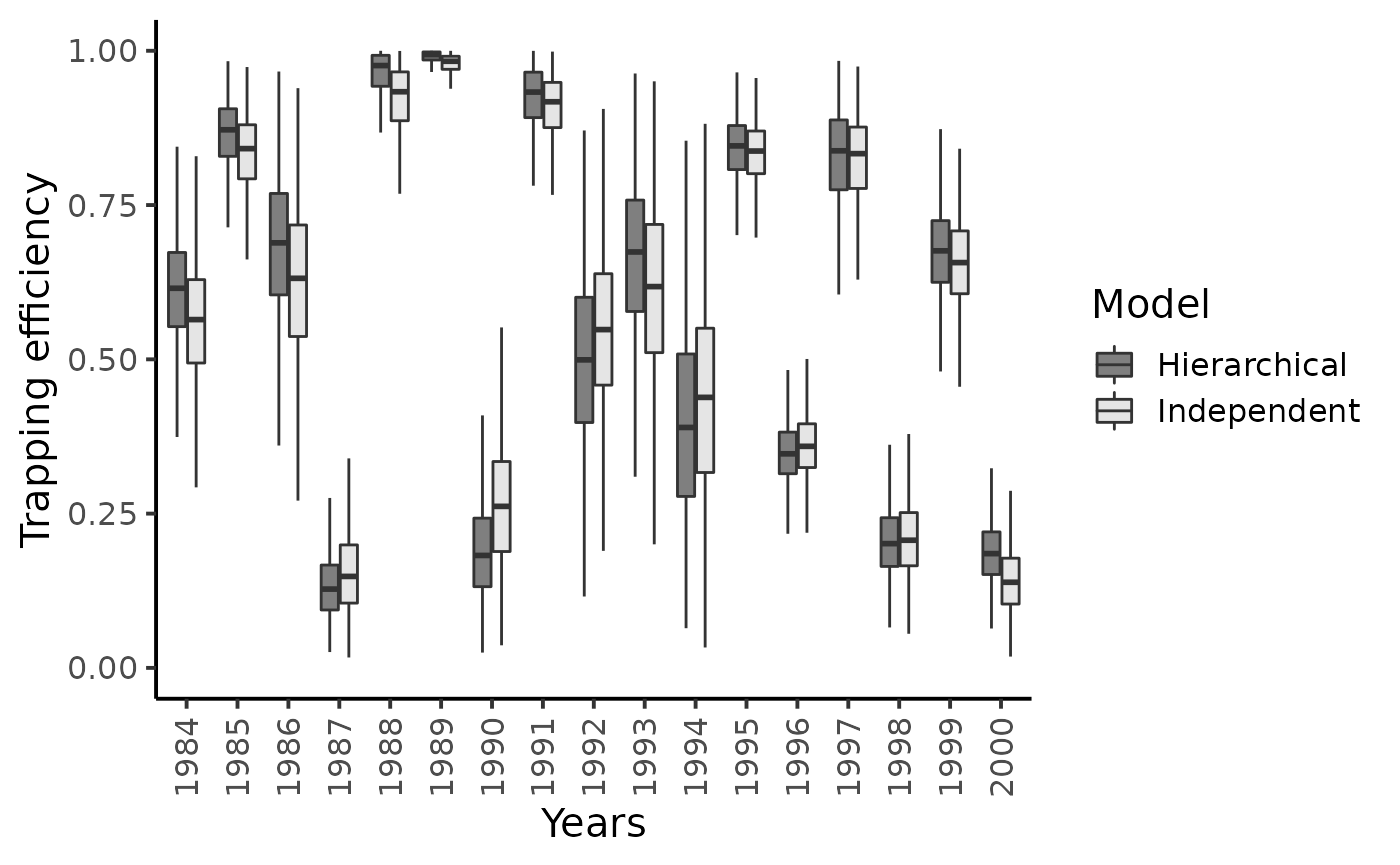dfl_ind %>% bind_rows(dfl_hier) %>%
filter(str_detect(parameter, "^pi2\\.")) %>%
mutate(Years = as.factor(seq(1984,2000)[as.integer(str_sub(parameter, 5))])) %>%  # 5 = length("pi2.") + 1
ggplot() +
aes(x = Years, y = value, fill = Model ) +
geom_boxplot(outlier.shape = NA, outlier.size = .25) +
scale_x_discrete(guide = guide_axis(angle = 90)) +
ylim(c(0,0.75)) +
scale_fill_manual(values = c("gray50", "gray90")) +
ylab("Recapture efficiency") + xlab("Years") +
theme_classic(base_size = 15L)
#> Warning: Removed 14 rows containing non-finite values (stat_boxplot).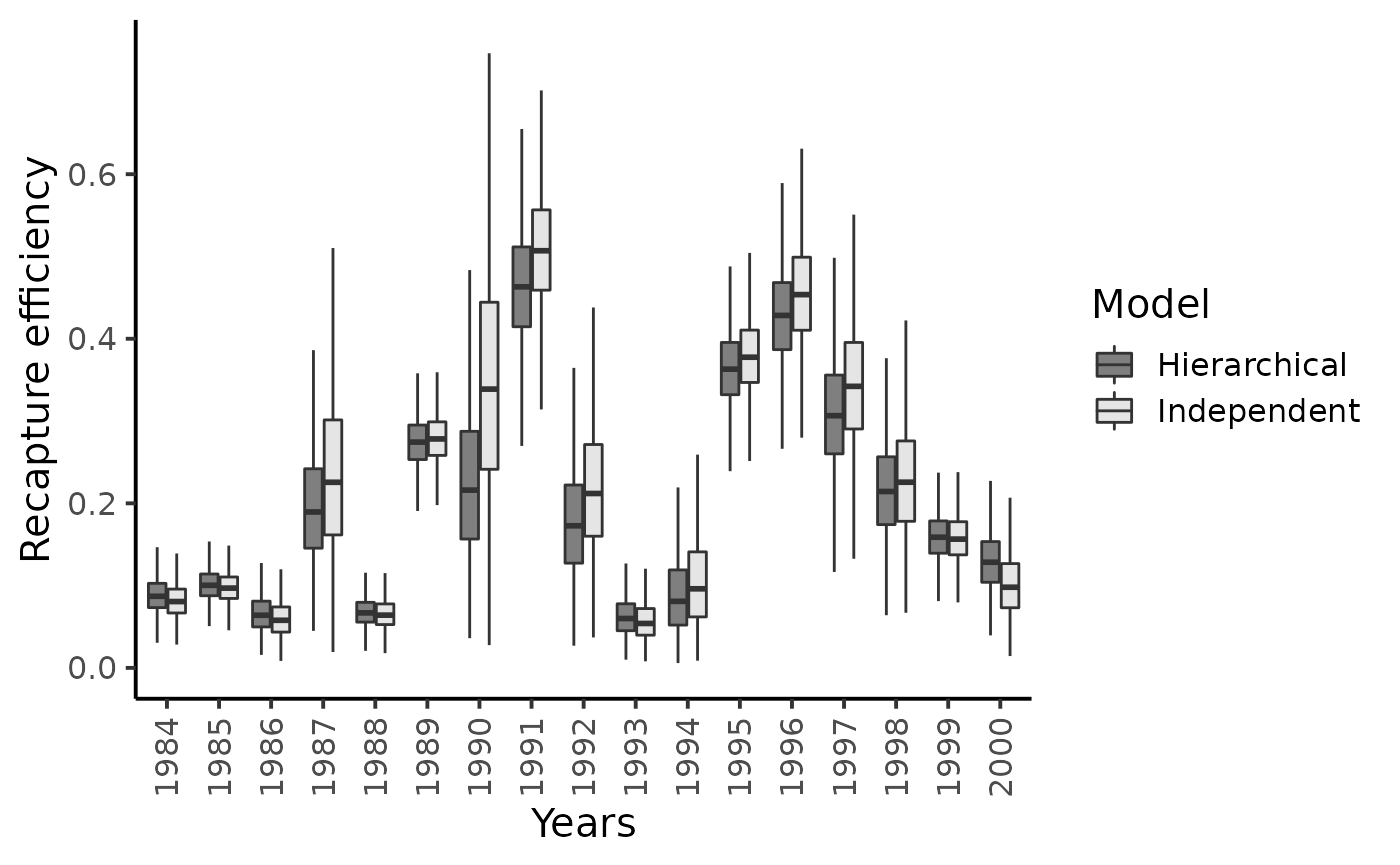dfl_ind %>% bind_rows(dfl_hier) %>%
filter(str_detect(parameter, "^nu\\.")) %>%
mutate(Years = as.factor(seq(1984,2000)[as.integer(str_sub(parameter, 4))])) %>%  # 4 = length("nu.") + 1
ggplot() +
aes(x = Years, y = value, fill = Model ) +
geom_boxplot(outlier.shape = NA, outlier.size = .25) +
scale_x_discrete(guide = guide_axis(angle = 90)) +
ylim(c(0,1000)) +
scale_fill_manual(values = c("gray50", "gray90")) +
ylab("Spawners") + xlab("Years") +
theme_classic(base_size = 15L)
#> Warning: Removed 53 rows containing non-finite values (stat_boxplot).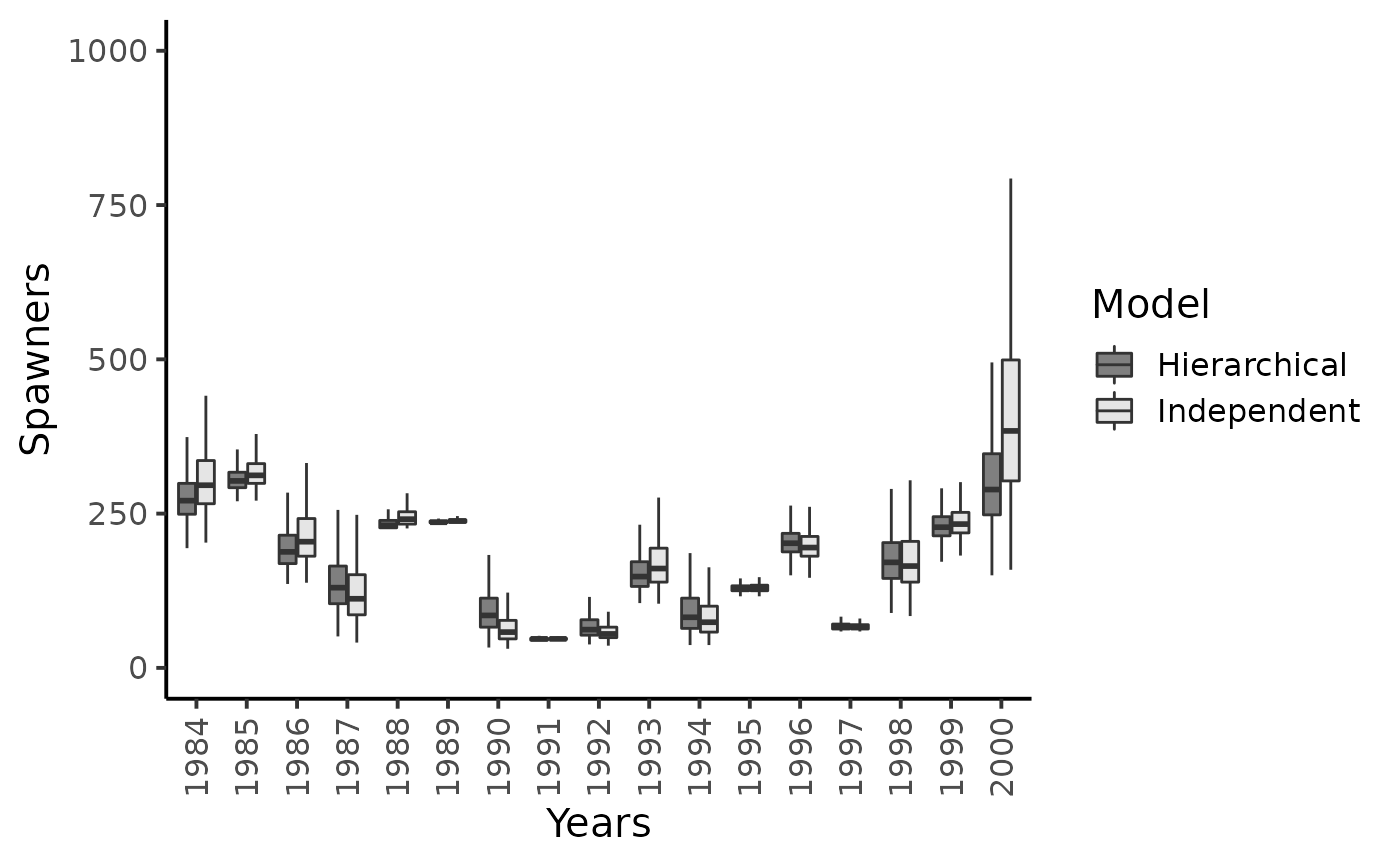Results highlight that hierarchical modeling has no effect on the inferences on the capture efficiencies but gratly improve posterior inferences of psawners migrating back to the Oir River.

Posterior mean values of the capture probabilities $$\pi_{t}^{1}$$ do not seem to shrink much toward their overall grand mean and the recapture probabilities $$\pi_{t}^{2}$$’s are only slightly subjected to the shrinkage effect. There remains a lot of between-year variability in the experimental conditions at the Cerisel trapping facility.

Conversely, the hierarchical structure hypothesized on $$\nu_{t}$$’s strongly reduces the skewness and uncertainty in the estimation of the number of spawners. The grey boxplots clearly point out that the most precise inferences are obtained under the hierarchical model, especially for the years with sparse CMR data, i.e., low number of marked released or, more importantly, low number of recaptures of previously marked fish yield (e.g., years $$1987$$, $$1990$$, $$1994$$ and $$2000$$). For this latter year, the upper bounds of the $$95\%$$ Bayesian credibility intervals obtained with the model assuming independence between years appears unrealistically high given the size of the Oir River and the available knowledge on the biology and ecology of Atlantic salmon as exemplified for year $$2000$$.

dfw_ind %>% bind_rows(dfw_hier) %>%
ggplot(aes(x = nu.17, linetype = Model)) +
geom_density() +
xlim(c(0, 1000)) +
xlab("Spawners") + ylab("") +
theme_classic(base_size = 15L)
#> Warning: Removed 53 rows containing non-finite values (stat_density).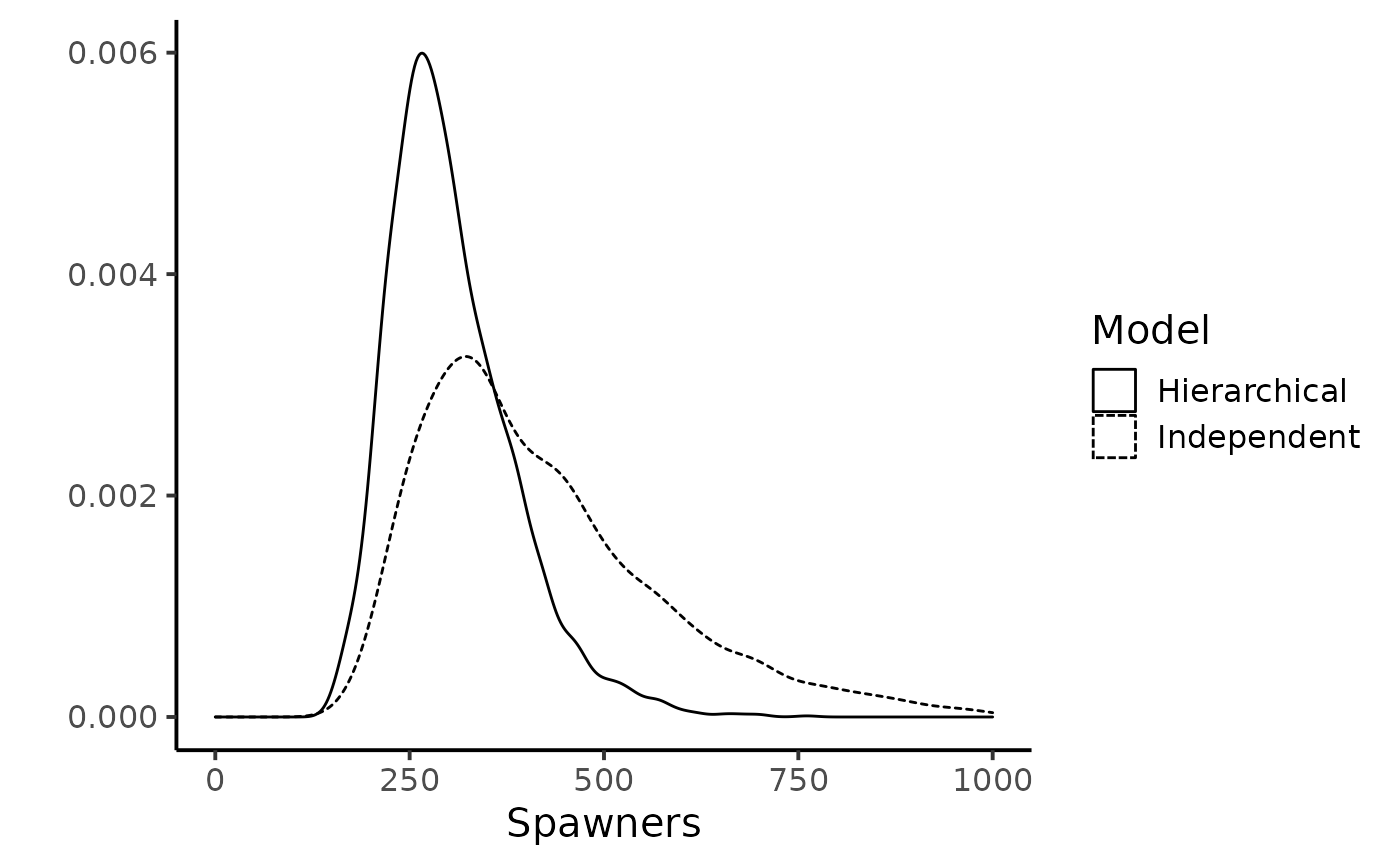#### Posterior predictive of the hierarchical model

summarise_draws(as_draws_df(posterior_sample_hier),
default_summary_measures()) %>%
filter(str_detect(variable, "pred"))
#> # A tibble: 3 × 7
#>   variable    mean  median      sd    mad      q5     q95
#>   <chr>      <dbl>   <dbl>   <dbl>  <dbl>   <dbl>   <dbl>
#> 1 nu_pred  181.    157     116.    96.4   45      395.
#> 2 pi1_pred   0.595   0.636   0.299  0.379  0.0746   0.990
#> 3 pi2_pred   0.209   0.182   0.142  0.136  0.0313   0.484
par.labs <- c("Spawners", "Trapping efficiency",
"Recapture efficiency")
names(par.labs) <- c("nu_pred", "pi1_pred", "pi2_pred")
dfl_hier %>%
filter(str_detect(parameter, "pred")) %>%
ggplot(aes(x = value)) +
xlab("")+ ylab("") +
facet_wrap( ~ parameter,
scales = "free",
labeller = labeller(parameter = par.labs)) +
geom_density() +
theme_classic(base_size = 15L)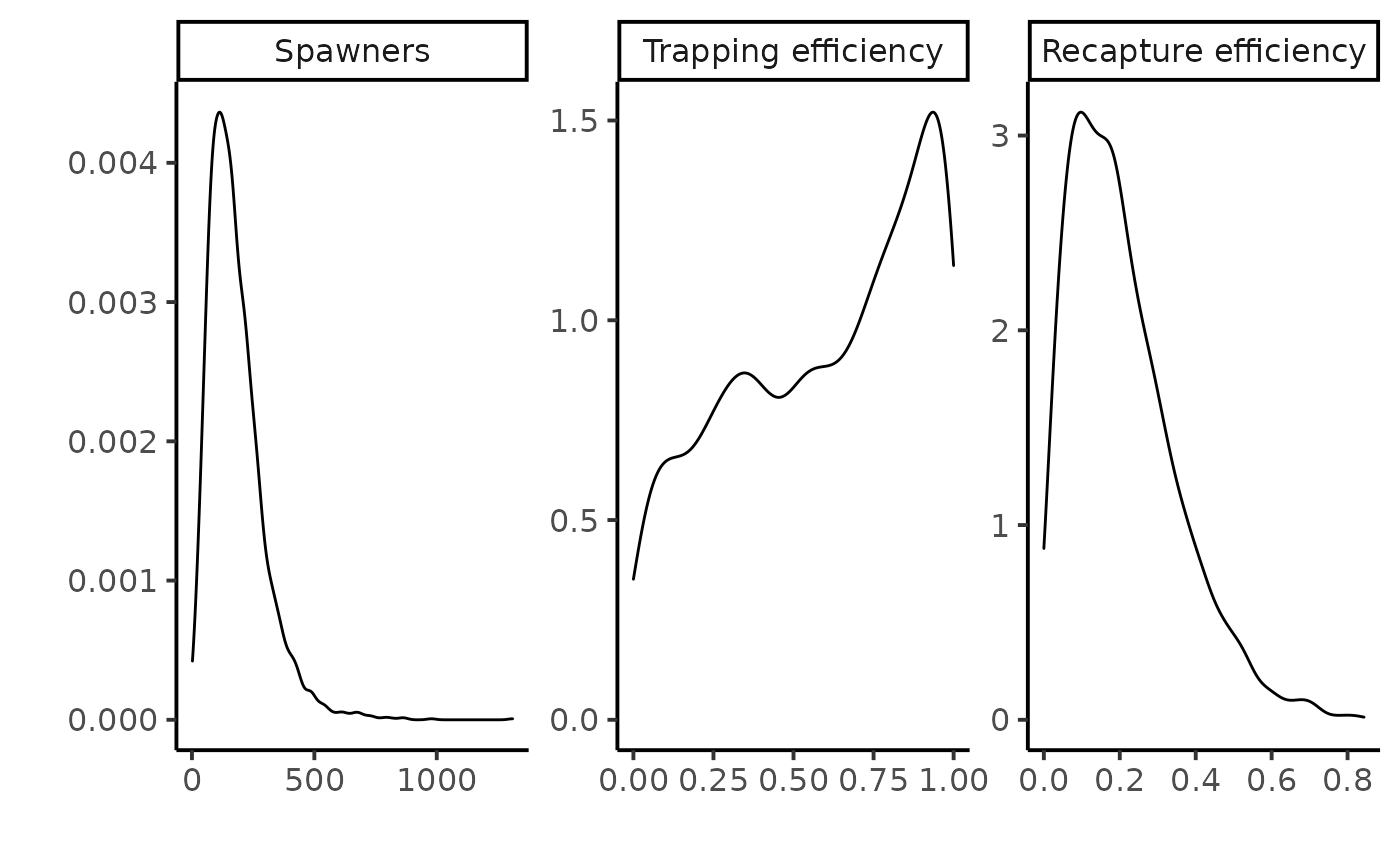A straightforward result of the hierarchical model are the posterior predictive distributions of the trapping or recapture efficiencies and of the number of returns , denoted $$[\pi^{1,new}|data_{1984:2000}]$$ and $$[\pi^{2,new}|data_{1984:2000}]$$, and $$[\nu^{new}|data_{1984:2000}],$$ respectively. The posterior predictive of the recapture efficiency and of the returns are informative distributions. Thus, the data of all years combined allow discarding a priori the possibility of very high trapping efficiency (i.e., greater than 0.5) or high spawner population size (i.e., greater than a thousand) in any additional year.

In-depth sensitivity analyses and another observation model for the recaptures can be found in Rivot and Prévost (2002).

Rivot, E., and E. Prévost. 2002. “Hierarchical Bayesian Analysis of Capture-Mark-Recapture Data.” Canadian Journal of Fisheries and Aquatic Sciences 59: 1768–84.